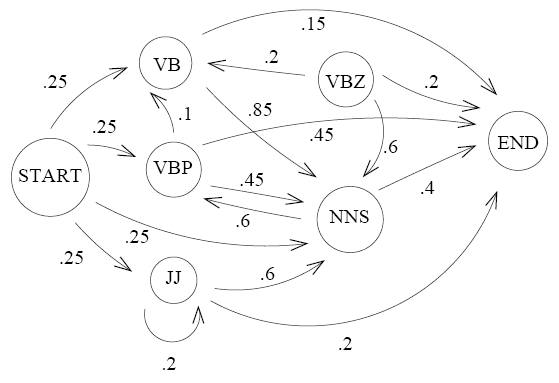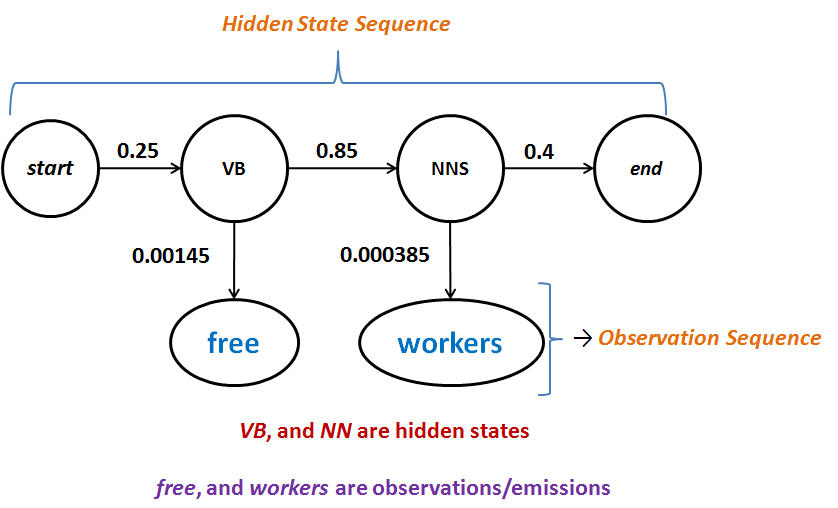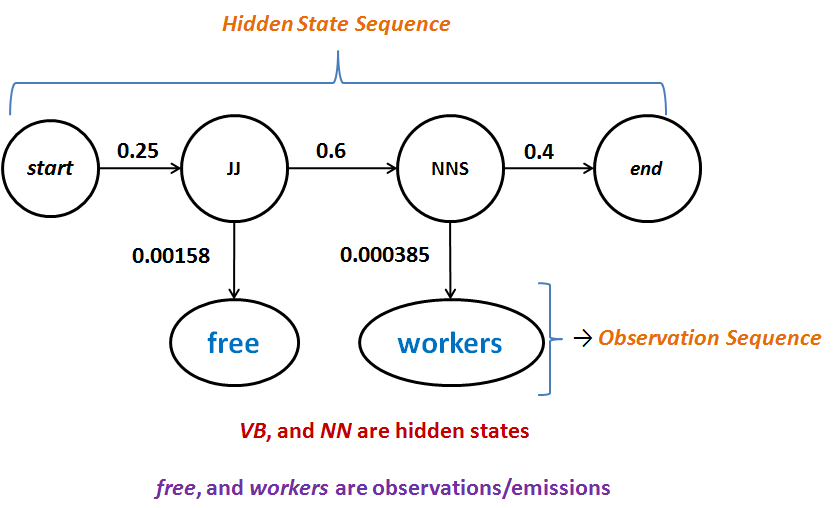Please visit, subscribe and share 10 Minutes Lectures in Computer Science

## POS Tagging using Hidden Markov Model - Solved Exercise

Question:
Consider the HMM given below to solve the sequence labeling problem of POS tagging. With that HMM, calculate the probability that the sequence of words “free workers” will be assigned the following parts of speech;
(a) VB NNS
(b) JJ NNS
HMM as stochastic automataEmission/Observation probabilities

 free workers JJ 0.00158 0 NNS 0 0.000385 VB 0.00145 0 VBP 0.00081 0 VBZ 0 0.00005

Solution:
(a) Here, the word sequence “free workers is tagged with tags VB NNS with the meaning to let the workers free. The HMM can be expressed as Bayes network for the given problem of finding the probability P(free workers, VB NNS) as follows;P(free workers, VB NNS)
= P(VB|start) * P(free|VB) * P(NNS|VB) * P(workers|NNS)
* P(end|NNS)
= 0.25 * 0.00145 * 0.85 * 0.000385 * 0.4
= 0.0000000475
= 4.75 * 10-8

(b) Here, the word sequence “free workers is tagged with tags JJ NNS to mean that the workers those work for free. The HMM can be expressed as Bayes network for the given problem of finding the probability P(free workers, JJ NNS) as follows;P(free workers, JJ NNS)
= P(JJ|start) * P(free|JJ) * P(NNS|JJ) * P(workers|NNS)
* P(end|NNS)
= 0.25 * 0.00158 * 0.6 * 0.000385 * 0.4
= 0.0000000365
= 3.65 * 10-8

*************